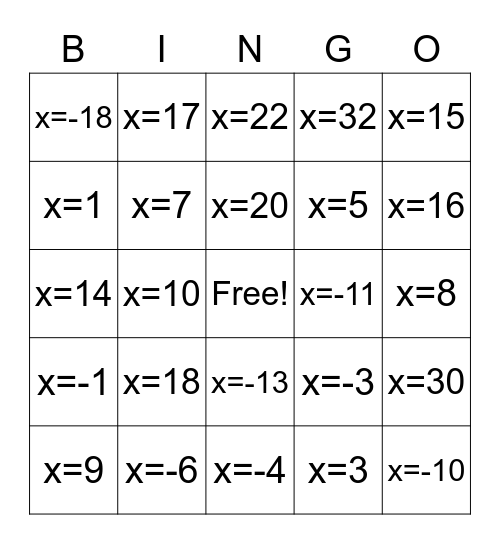# Two-Step Equations BINGOThis bingo card has a free space and 27 words: x=-3, x=12, x=5, x=-4, x=1, x=22, x=17, x=-1, x=-11, x=30, x=14, x=32, x=9, x=-6, x=16, x=-2, x=7, x=20, x=18, x=-18, x=8, x=3, x=10, x=-10, x=-13, x=15 and x=-7.

## Play Online